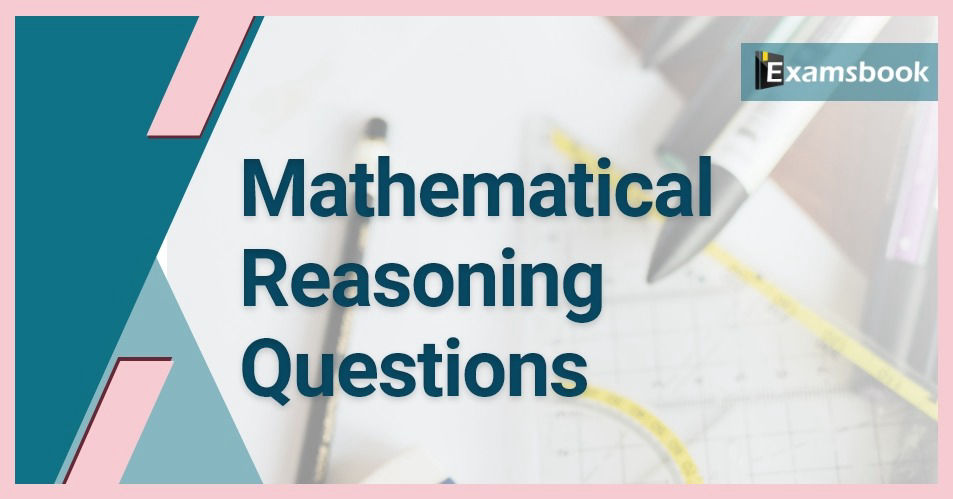• Save

# Mathematical reasoning questions with answers for Competitive ExamsMathematical reasoning questions are most important for competitive exams. So, don't ignore your mistakes while solving mathematical reasoning questions in your preparation. Students should try to attempt these mathematical reasoning questions with answers. Let's solve mathematical reasoning questions exercise yourself for better understanding.

Preparation is the foundation of all competitive exams but it will be easy if you will do preparation with selective questions and answers. So keep practice with these Mathematical reasoning  questions and answers.

## Choose the Topics of Mathematical Reasoning:

 Maths Puzzles Questions with Answers Maths Questions and Answers for Bank Exams Mathematical questions and answers in Hindi Maths Logical Reasoning Questions with Answers Maths Inequality Questions for Bank Po Exams Time and Work Maths Questions

Also, Read Latest Current Affairs Questions 2023: Current Affairs Today

"Put your knowledge to the test and excel with our General Knowledge Mock Test and Current Affairs Mock Test!"

## Mathematical Reasoning

Q :

Which of the following symbols should be inserted in place of question mark (?) respectively (in the same order from left to right) in order to complete the given expression in such a manner that ‘U > S’, ‘Q < T’ and ‘S ≤ N’ definitely holds true?
U > T ? D ≥ S ? Q ? N

(A) >, >, <

(B) >, =, <

(C) ≥, =, ≤

(D) >, =, ≤

(E) >, =, ≤

Q :

Which of the following symbols should be placed in the blank space in order to complete the given expression in such a manner that ‘X > I’ definitely true?
A = R ≥ I ? T = N ≤ M = X

(A) ≥

(B) =

(C) <

(D) >

(E) ≤

Q :

Which of the following symbols should be placed in the blank spaces respectively (in the same order from left to right) in order to complete the given expression in such a manner that “D < A” definitely holds True?
A __ B __ C __ D

(A) >, ≥, <

(B) ≤,

(C) ≥, =, <

(D) ≥, =, >

(E) None of these

Q :

Select the correct combination of mathematical signs to sequentially replace the * signs and balance the given equation.
21 * 3 * 36 * 2 * 23 = 68

(A) ÷ − × +

(B) ÷ × + −

(C) + × − ÷

(D) × − ÷ +

Q :

If + means −, − means ×, × means ÷, and ÷ means +, what will be the value of the following expression?
5 ÷ 5 + 5 – 10 × 10 = ?

(A) 15

(B) 4

(C) 5

(D) 10

Q :

By interchanging the given two signs and numbers which of the following equation will be correct?
+ and –, 7 and 6

(A) 4 × 7 + 6 – 3 ÷ 1 = 20

(B) 8 × 7 + 5 ÷ 1 – 6 = 17

(C) 7 – 6 × 3 + 4 ÷ 1 = 8

(D) 7 × 2 – 6 + 4 ÷ 2 = 13

Q :

Select the correct combination of mathematical signs to sequentially replace the # signs and to balance the given equation.
[{(44 # 38) # (2 # 5)} # (4 # 2)] # 5 # 10

(A) ×, ÷, ×, ×, −, +, =

(B) ×, ̶ , +, ×, ÷, ×, =

(C) ̶ , +, ×, ×, ÷, ×, =

(D) ̶ , +, ×, ÷, ×, ×, =

Q :

Select the correct combination of mathematical signs to sequentially replace the * signs and to balance the given equation.
25 * 2 * 220 * 11 * 43

(A) +, −, ×, =

(B) +, −, ÷, =

(C) +, +, ÷, =

(D) −, +, ÷, =

Q :

By Interchanging the given two numbers which of the following equation will be correct?
1 and 7

(A) 7 × 2 + 1 – 5 = 6

(B) 1 × 2 – 7 + 8 ÷ 2 = 16

(C) 7 + 1 × 2 – 6 ÷ 3 = 13

(D) 7 + 6 ÷ 3 – 5 × 1 = – 10

Q :

If ‘+’ means ‘÷’, ‘ ̶ ’ means ‘+’, ‘×’ means ‘ ̶ ’ and ‘÷’ means ‘×’, what will be the value of the following expression?
[{(32 × 20) - (2 ÷ 3)} + (2 - 4)] ÷ 3

(A) 8

(B) 10

(C) 9

(D) 6